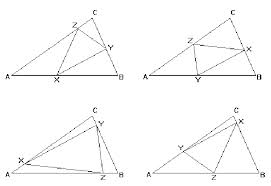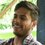# Geometry ProblemsImage

My Questions are:

$$\bullet$$ Find the minimum perimeter of triangle XYZ.

$\bullet$ Find the maximum perimeter of triangle XYZ.

$\bullet$ Find the minimum perimeter of triangle XYZ when the outer triangle has maximum perimeter.

$\bullet$ Find the maximum perimeter of triangle XYZ when the outer triangle has maximum perimeter.

$\bullet$ Find the minimum perimeter of triangle XYZ when the outer triangle has minimum perimeter.

$\bullet$ Find the maximum perimeter of triangle XYZ when the outer triangle has minimum perimeter.

Details and assumptions (In all cases)

$\bullet$ Inner triangle XYZ must touches all sides outer triangle ABC

$\bullet$ Outer triangle is an acute angled triangle.

$\bullet$ Circum-radius of outer Triangle is R=1

I posted a similar question earlier which is was a blunder. Since when I trying to answer the question which was asked by my friend that is:

" What is condition for minimum possible perimeter of inner triangle XYZ which inscribed in the outer triangle ABC when there is no constraint ?? "

Then I discover that XYZ must be orthic triangle and he tells me that I'm correct.

So my next work is that to calculate that minimum perimeter. But I'm unable to solve it at my own. So I posted a Note on this question

But from that question my curiosity is increased and I decided that if I fixed 'R', then the perimeter of Triangle XYZ should be maximum if it is an orthic triangle By Jenson's Inequality. So I posted that question

Which is later on found to be wrong.

These are such question that are coming in my mind. Please try to solve all of them and then post your solutions please!

And If you have any more such type of questions then please share with us.

Thanks!Note by Deepanshu Gupta
6 years, 7 months ago

This discussion board is a place to discuss our Daily Challenges and the math and science related to those challenges. Explanations are more than just a solution — they should explain the steps and thinking strategies that you used to obtain the solution. Comments should further the discussion of math and science.

When posting on Brilliant:

• Use the emojis to react to an explanation, whether you're congratulating a job well done , or just really confused .
• Ask specific questions about the challenge or the steps in somebody's explanation. Well-posed questions can add a lot to the discussion, but posting "I don't understand!" doesn't help anyone.
• Try to contribute something new to the discussion, whether it is an extension, generalization or other idea related to the challenge.
• Stay on topic — we're all here to learn more about math and science, not to hear about your favorite get-rich-quick scheme or current world events.

MarkdownAppears as
*italics* or _italics_ italics
**bold** or __bold__ bold
- bulleted- list
• bulleted
• list
1. numbered2. list
1. numbered
2. list
Note: you must add a full line of space before and after lists for them to show up correctly
paragraph 1paragraph 2

paragraph 1

paragraph 2

[example link](https://brilliant.org)example link
> This is a quote
This is a quote
    # I indented these lines
# 4 spaces, and now they show
# up as a code block.

print "hello world"
# I indented these lines
# 4 spaces, and now they show
# up as a code block.

print "hello world"
MathAppears as
Remember to wrap math in $$ ... $$ or $ ... $ to ensure proper formatting.
2 \times 3 $2 \times 3$
2^{34} $2^{34}$
a_{i-1} $a_{i-1}$
\frac{2}{3} $\frac{2}{3}$
\sqrt{2} $\sqrt{2}$
\sum_{i=1}^3 $\sum_{i=1}^3$
\sin \theta $\sin \theta$
\boxed{123} $\boxed{123}$

## Comments

There are no comments in this discussion.

×

Problem Loading...

Note Loading...

Set Loading...